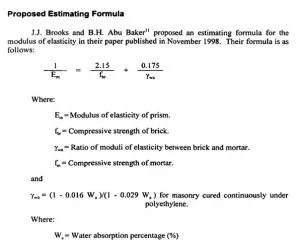# Relation between Masonry Compressive Strength and Modulus of Elasticity

Relation between Masonry Compressive Strength and Modulus of Elasticity

Following seven relations used by different researchers have been shown here.

1.Source: Book “Seismic Design of Reinforced Concrete and Masonry Buildings” By T. Paulay and M. J. N. Priestley

Relation

Concrete Masonry: Em = 1000f′m
Clay Brick Masonry: Em = 750f′m
Where
Em = Modulus of elasticity
f′m = Compressive Strength of Masonry

2.Source: “Uniaxial compressive strength and stiffness of field extracted and laboratory constructed masonry prisms”  By Ronald Lumantarna, David T. BiggsM. ASCE and Jason M. Ingham M. ASCE

The masonry Modulus of Elasticity (Em) is commonly calculated as the chord modulus of the linear part of the masonry compression stress-strain curve, which is typically defined to be between 5% and 33% of the ultimate masonry compressive strength (f’m) (ASTM 2003a; Drysdale et al. 1999). Alternatively, Gumaste et al. (2006) used the secant modulus at 0.25  to calculate the masonry Modulus of Elasticity. The relationship between masonry compressive strength and Modulus of Elasticity can be expressed as

Relation

Em = k f’m

where k is a constant that varies from one recommendation to another. The MSJC code (2002) and FEMA 306 (1999) from North America recommend that Em is equal to 700f’m  for modern masonry and 550f’m  for existing masonry respectively, whilst the Canadian masonry code (CSA 2004) suggests a slightly higher value of   Em= 850f’m  for modern masonry. Paulay and Priestley (1992) and Eurocode 6 (CEN 2005) suggest that Em is equal to 750f’m  and 1000f’m  respectively. In addition, Kaushik et al. (2007a) observed that there was a wide variation in the Modulus of Elasticity-compressive strength relationships of newly constructed Indian clay brick masonry, where the  values varied from 250f’m  to 1100f’m . This wide variation was also observed by Drysdale et al. (1999), who collected past experimental data and encountered  Em values ranging from 210f’m  to 1670f’m

3.Source: BS 5628

Relation

E = 900 f N/mm2

4.Source: Eurocode 6

Relation

E =1000 f N/mm2

5.Source: EXPERIMENTAL COMPRESSIVE STRENGTH AND MODULUS OF ELASTICITY OF MASONRY  By Ida Ayu Made Budiwati

Relation

E =1000 f N/mm2

6.Source: Design of Masonry for Buildings (Limit States Design) CSA S304.1-M94; Canadian Standards Association, 1994.

Relation

E =850 f

7.Source: “ The modulus of elasticity of Masonry” By J. Brooks and B.H. Abu BakarMasonry Compressive Strength and Modulus of Elasticity,  Masonry Compressive Strength and Modulus of Elasticity,  Masonry Compressive Strength and Modulus of Elasticity,  Masonry Compressive Strength and Modulus of Elasticity,  Masonry Compressive Strength and Modulus of Elasticity,  Masonry Compressive Strength and Modulus of Elasticity,  Masonry Compressive Strength and Modulus of Elasticity,  Masonry Compressive Strength and Modulus of Elasticity,  Masonry Compressive Strength and Modulus of Elasticity,